### Home > MC1 > Chapter 6 > Lesson 6.1.4 > Problem6-40

6-40.
1. . The data given in the table at right compares the weight of bags of quarters with the number of quarters in the bag. Homework Help ✎

Number of quarters

Weight (grams)

0

1

2

3

4

5

30

6

7

8

48

9

10

1. Copy and complete the table.

2. Graph the data in the table.

3. How much does each quarter weigh? Show how you know.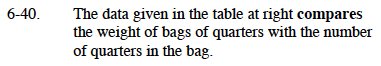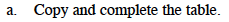If 5 quarters weigh 30 grams, how many grams does one quarter weigh? Use this data to complete the table.

Your completed table should look like the one to the right.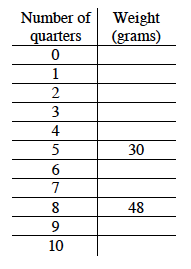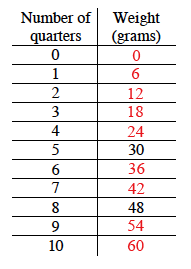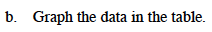It would be smart to label the horizontal x-axis ''Number of Quarters'' and your vertical y-axis ''Weight (grams).''

Can you scale your axis? For a reminder on how to do this, refer to problem 4-134.

For help on how to plot your coordinate points, refer to problem 5-44. Remember, if you were to record your coordinate points, they would read (Number of Quarters, Weight).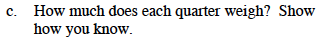Looking at your table, can you tell how much one quarter weighs? Remember to show how you know!